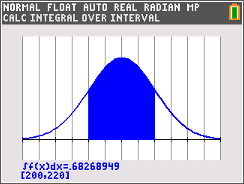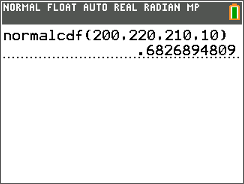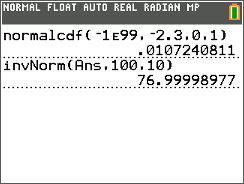# Activities

••• ##### Subject Area

• Math: Statistics: Normal Distributions

• ##### Author9-12

45 Minutes

• ##### Device
• TI-84 Plus
• TI-84 Plus Silver Edition
•TI-84 Plus C Silver Edition
•TI-84 Plus CE

## Percentiles & Z-Scores#### Activity Overview

Students calculate percentiles, z-scores, and probabilities using normal distributions.

#### Key Steps

•The activity begins with a review of the properties of normal curves along with a visual representation of the corresponding percentages. The worksheet then introduces z-scores and percentiles.

•Students are given a range of x-values and asked to find the probability (or percent) of data that fall within the interval. To find the answer, they will first use a graph and then follow up with numerical calculations.

•In the last two parts, students are to find the corresponding x-value given a percentile and then given a z-score and they are asked to find the corresponding percentile and x-value.